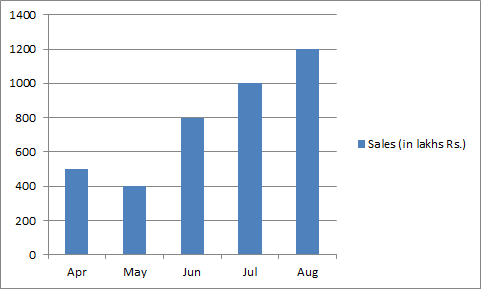# Data Interpretation - Bar Graph

>>>>>>>>Bar Graph

Instructions:

Study the following graph carefully and answer the question16-20  given below• A

May to July• B

May to August• C

June to August• D

None of theseInstructions:

Study the following graph carefully and answer the question16-20  given below

• A

April• B

May• C

June• D

July• Option : C
• Explanation : Proportion of the sales in June to that in May = 800/400=2

July to that in June = 100/800 = 5/4 = 1.25

August to that in July = 1200/1000= 6/5 = 1.20

Instructions:

Study the following graph carefully and answer the question16-20  given below

• A

2.5• B

3.00• C

3• D

None of theseInstructions:

Study the following graph carefully and answer the question16-20  given below

• A

800• B

700• C

1200• D

400Instructions:

Study the following graph carefully and answer the question16-20  given below

• A

600• B

650• C

780• D

620• Option : C
• Explanation : Average monthly sales = (500+400+800+1000+1200) / 5 = 780
Related Quiz.
Bar Graph